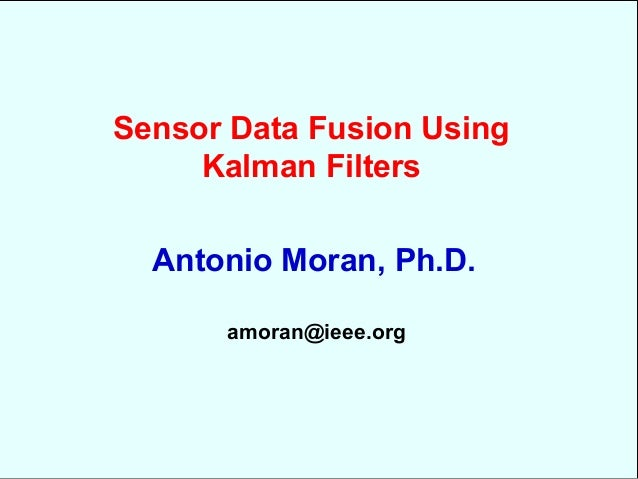Successfully reported this slideshow.
We use your LinkedIn profile and activity data to personalize ads and to show you more relevant ads. You can change your ad preferences anytime.Upcoming SlideShare
Loading in …5
×
• Full Name
Comment goes here.

Are you sure you want to Yes No
Your message goes here• Be the first to comment

### Data fusion with kalman filtering

1. 1. Sensor Data Fusion UsingKalman FiltersAntonio Moran, Ph.D.amoran@ieee.org
2. 2. Kalman FilteringEstimation of state variables of a systemfrom incomplete noisy measurementsFusion of data from noisy sensors to improvethe estimation of the present value of statevariables of a system
3. 3. Kalman FilteringGiven a Systemx = A x + B u + w.y = Cx + nwherex = nx1 state vectory = mx1 measurement vectorw = nx1 process noisesystem disturbancen = mx1 measurement noiseQ = w covariance matrixR = n covariance matrixSystemuwnyw and n are white gaussiannoises
4. 4. Kalman FilteringProblem Definition^Find an estimate x of state vector x fromincomplete and noisy measurement vector y
5. 5. Kalman FilteringSystemx = A x + B u + w.y = Cx + nKalman Filter (Estimator)x = A x + B u + L (y – C x).^^w and n are not included. Theirpresent values are unknown.L: filter gain. nxm matrix.Filter gain L ensures that the estimation error econverges to zerolim e(t) 0t ∞e = x - x^
6. 6. Kalman FilteringSystem.uwnyState xEstimator.State x^x^
7. 7. Kalman FilteringComputing the Filter Gain Lx = A x + B u + L (y – C x).^^L = S CTR-1S is a positive matrix solution of the Riccati equation:A S + SAT- S CTR-1CTS + Q = 0Matrixes A, C, Q and R are knownEstimator:
8. 8. Data Fusion Using Kalman FilterProblem FormulationFuse the noisy signals of three sensors toimprove the estimate of a variable (temperature,distance, position, etc.)
9. 9. Air TemperatureSensor 1Sensor 2Sensor 3Gaussian processmean value: 20std. dev.: 1.1Sensors noise with zero mean
10. 10. Sensor 1Sensor 2Sensor 3Sensors Noise and Biasstd. dev.: 0.8bias: 0.4std. dev.: 0.8bias: -0.4std. dev.: 0.8bias: 0.0
11. 11. Sensor Data Fusion with Kalman FilterSensor 1Sensor 2Sensor 3Sensor DataFusion withKalman FilterImprovedEstimation ofMeasured Variable
12. 12. Kalman FilteringSystemx = A x + B u + w.y = Cx + nx = w.y1 = x + n1y2 = x + n2y3 = x + n3x = air temperatureChanges in temperature aremodeled as a gaussian processSensor 1Sensor 2Sensor 3Noisy measurementsDetermining matrixes:A = 0 B = 0 C =111Q = 1.21 R =0.64 0 00 0.64 00 0 0.64
13. 13. Estimation Using One SensorThe Kalman filter significantly reduces the noisebut does not eliminate the biasFirst sensor with noise and bias
14. 14. Estimation Using Two SensorsSensor fusion through Kalman filteringsignificantly improves the estimation reducingthe effect of sensor noise and biasFirst and Second sensors with noise and bias
15. 15. Estimation Using Three SensorsNo significantly improvement with the third sensor(respect to when using first and second sensors)Three sensors. Third sensor only with noise
16. 16. Estimation ErrorHigher error when using only the first sensor with bias.e = x - x^Lower error when using two and three sensors.
17. 17. Estimation ErrorMean Value Standard Deviatione = x - x^First SensorFirst and SecondSensorsThree Sensors-0.00035 0.082111-0.00051 0.072695-0.39896 0.097506Higher estimation error when using only the firstsensor (noise and bias).Estimation error significantly reduces with two andthree sensors. Bias is almost eliminated.
18. 18. Estimator Performace for Non-Gaussian ProcessesThe estimator performs well even if the processis not gaussian.Temperature changes behave as a uniform(non-gaussian) process with zero mean
19. 19. ConclusionsSensor data fusion can be easily implemented usingKalman filters.For filter design, changes in temperature are modeled as agaussian process. However, the filter performs well evenwhen used in other probabilistic processes.Sensor fusion through Kalman filtering significantlyimproves the on-line estimation reducing the effect ofsensor noise and bias.Research goes on for the simultaneous estimation ofseveral variables.